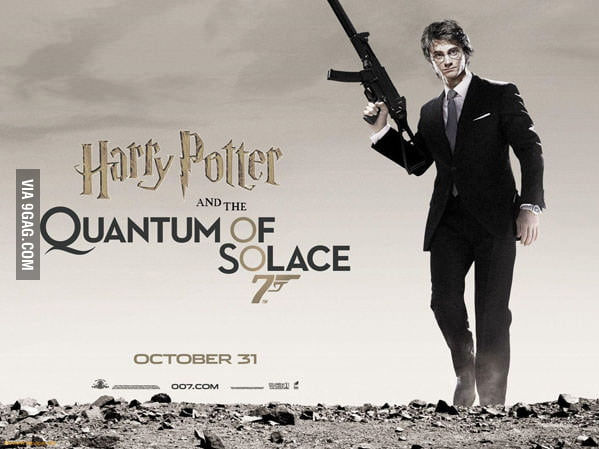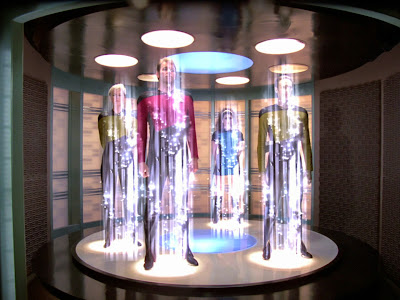## Saturday, July 15, 2017

### Quantum teleportation is less magical than both Harry Potter apparating, Star Trek beaming

Popularizers of science often want the laymen feel excited. So they say "this experiment is amazing", "this experiment is magical". At the end, I think that this hype creates more damages than benefits.

Four months ago, we were being persuaded that the Chinese have constructed a quantum radar, something that can inform you about an airplane without any actual reflection of any radiation from the airplane. This is obviously impossible by locality, whether you use any quantum subtleties or not.Right now, we're told about another wonderful achievement by the Chinese. They have succeeded in teleporting a particle to the outer space. It's basically the same topic – about the same Chinese and about the same transmission of quantum information to the outer space. There are stories everywhere. NPR chose the title
Beam Me Up, Scotty ... Sort Of. Chinese Scientists 'Teleport' Photon To Space
Brian Greene was asked whether the Chinese achievement is closer to Star Trek's "beaming" or Harry Potter wizards' "apparating". He answered that it's "somewhere in between" Harry Potter and Star Trek. He was mostly joking except that most readers and listeners won't understand in what sense he was joking – and many of them won't understand that he was joking at all. And it seems that this misinterpretation is really intentional.

There is nothing illogical, magical, or non-local about quantum teleportation. And there is nothing about quantum teleportation that would become significantly more difficult if the distance between the "parts of the lab" grows bigger.

What is quantum teleportation? It's not a real transmission of "general matter". It's just a transmission of quantum information. The quantum information may have many forms but the simplest toy model almost always involves one qubit.

OK, what is a qubit? It's the information that can be carried by a 2-state Hilbert space, e.g. the electron's spin. The general state of the electron spin is$\ket\psi = \alpha\ket\uparrow + \beta \ket\downarrow, \quad \alpha,\beta\in\CC$ Classically, the spin would objectively be up (=0) or down (=1), so there would be one bit of information. Quantum mechanically, things may be uncertain, $$|\alpha|^2$$ and $$|\beta|^2$$ are the probabilities – we should assume $$|\alpha|^2+|\beta|^2=1$$ – and on top of the separation of "one" into these two probabilities, the relative phase between $$\alpha,\beta$$ matters, too. The overall phase change of both $$\alpha,\beta$$ is unphysical, however.

How does Alice send the qubit to Bob? Well, if the qubit is carried by one electron, she just sends the whole electron. The electron will carry the same quantum information during the whole trip. Is there a different way that doesn't need to transfer the quantum information directly? Yes. It's enough if there were some quantum entanglement created in advance; and if one transfers some classical information even though you will be able to transmit quantum information.

How does it work? Let's first look at the classical toy model of the same situation.

A source AB of classical bits sends bits to two places, Alice's place and Bob's place. It can send either 00 or 01 or 10 or 11. Let's assume that AB sends 00 to both places. It's enough to assume that it's always AB=00.

Now, Alice wants to transfer the bit C which is either 0 and 1. In this case, we really don't know what the value is. She makes a combined measurement of AC, namely a measurement of "A XOR C". Because A=0, as we said in the previous paragraph, "A XOR C" is simply equal to C. She finds out the result of this "A XOR C" and sends the classical result of this measurement to Bob. Bob has his bit, B, but he can change it to the value of "A XOR C" that he heard from Alice: if Alice says "zero", he doesn't do anything. If Alice says "one", Bob negates his bit from "zero" to "one". At the end of the operation, Bob's bit B is equal to what Alice's C was to start with.

It was a talkative way to copy one damn bit, wasn't it? I formulated it in this awkward way because a straightforward – but somewhat more contrived – generalization exists that transmits C which is a qubit.

Let's redo the operation above in the fully quantum setup.

There is a source of entangled particles AB. Let's assume that they're in the state$|\Phi^+\rangle_{AB} = \frac{1}{\sqrt{2}} (|0\rangle_A \otimes |0\rangle_{B} + |1\rangle_A \otimes |1\rangle_{B})$ You can see that the probability is 100% that the $$\sigma_z$$-measurements of both qubits end up with the same result. We also had to choose the relative phase or sign of the two terms. Fine. This source of entangled qubits AB is split, A is sent to Alice's place, B is sent to to Bob's place.

Alice wants to "beam" a qubit C to Bob. At this moment, we should realize that the state of all three qubits is$|\Phi^+\rangle_{AB} \otimes |\psi\rangle_C = \quad\quad\quad\tag{2017.7}$$=\frac{1}{\sqrt{2}} (|0\rangle_A \otimes |0\rangle_B + |1\rangle_A \otimes |1\rangle_B)\otimes (\alpha |0\rangle_C + \beta|1\rangle_C).$ Now, Alice will make a measurement that is the quantum counterpart of "A XOR C" in my classical story. She will make a combined measurement on the two qubits A,C which she has access to which will produce one of the 4 results in this orthonormal basis of the 2-qubit Hilbert space. These four states are $$\ket{\Phi^\pm}$$ and $$\ket{\Psi^\pm}$$. Phi is composed of 00 and 11, Psi is composed of 01 and 10, and the $$\pm$$ sign determines the relative sign between the two terms.

Just to make sure that we write something newer, let's invert the relationship between the two bases, the basis of 00,01,10,11, and the basis containing $$\ket{\Phi^\pm}$$ and $$\ket{\Psi^\pm}$$. The former basis vectors may be written in terms of the latter as:$\displaystyle |0\rangle \otimes |0\rangle = \frac{1}{\sqrt{2}} (|\Phi^+\rangle + |\Phi^-\rangle),\\ \displaystyle |0\rangle \otimes |1\rangle = \frac{1}{\sqrt{2}} (|\Psi^+\rangle + |\Psi^-\rangle),\\ \displaystyle |1\rangle \otimes |0\rangle = \frac{1}{\sqrt{2}} (|\Psi^+\rangle - |\Psi^-\rangle),\\ \displaystyle |1\rangle \otimes |1\rangle = \frac{1}{\sqrt{2}} (|\Phi^+\rangle - |\Phi^-\rangle).$ Let us substitute this for the states 00,01,10,11 of the AC qubits in the expression (2017.7); just try to appreciate how much smarter you have gotten if you can actively use equations such as (2017.7). The state may be rewritten as\begin{align} |\Phi^+\rangle_{AB} \ \otimes\ | & \psi\rangle_C = \\ \frac{1}{2} \Big \lbrack \ & |\Phi^+\rangle_{AC} \otimes (\alpha |0\rangle_B + \beta|1\rangle_B) \\ \ + \ & |\Phi^-\rangle_{AC} \otimes (\alpha |0\rangle_B - \beta|1\rangle_B) \\ \ + \ & |\Psi^+\rangle_{AC} \otimes (\beta |0\rangle_B + \alpha|1\rangle_B) \\ \ + \ & |\Psi^-\rangle_{AC} \otimes (\beta |0\rangle_B - \alpha|1\rangle_B) \Big \rbrack . \\ \end{align} Excellent. Now, Alice has obtained one of the four results of her measurements, either $$\Phi^\pm$$ or $$\Psi^\pm$$, of the AC qubit pair. This result makes one of the four terms above relevant. Consequently, the wave function for the remaining qubit B also collapses to one of the four superpositions above. And for each of the 4 forms of the state, there is always a way to manipulate this qubit B – exchanging 0 and 1 and/or changing the relative sign in between the two terms – that ends up with the state of the B qubit in $$\alpha |0\rangle_B + \beta|1\rangle_B$$, i.e. in the original state of the qubit C.

So the qubit C in Alice's place had some quantum state and it was transferred to the state of qubit B that was in Bob's place. We needed previously transmitted entangled AB qubit pairs; and we needed Alice to inform Bob about the result of a classical measurement – one of the four options $$\Phi^\pm$$ or $$\Psi^\pm$$.That's it. It's quantum teleportation. It's not too different from simply sending the particle with the qubit C from one place to another. Alice still needs to send some information – which can't be done faster than light in any case – and Alice, Bob still need a path for sending entangled/quantum particles that go all the way from A to B – although the direction that is used is from "the AB source at the center to either A or B".

But regardless of the complex technicalities, quantum teleportation must be considered to be just one of the specific generalizations of the simple procedure of copying one bit of information that I started with. It's a generalization that can remember the two complex amplitudes. But because quantum cloning is impossible, the original state of the qubit C was destroyed by Alice's combined measurement of the AC qubit pair.

Aside from these technicalities – that we're talking about qubits that may be in superpositions, that may be manipulated by a more complex set of "unitary transformations" etc. – the quantum teleportation is morally nothing else than a version of a simple copying of one (quantum) bit. What Harry Potter's colleagues and Star Trek charlatans were doing was less elementary.

I think it's correct to say that the hype has a "wrong sign". The media are telling you that quantum mechanics allows you to do incredible things – such as those from the children's or sci-fi books and films – easily. But the actual message of the explanation above is that even seemingly trivial procedures – such as the copying of one bit of information – becomes subtle and hard in quantum mechanics. And quantum mechanics and theoretical physics in general is "cool" when it allows to make seemingly simple things hard or if you force you to be careful or hard-working. If someone isn't excited with this "imposed subtlety", then he simply doesn't like theoretical physics. He may like Harry Potter and Star Trek movies but those aren't the same thing as theoretical physics and from many perspectives, they have the opposite sign.